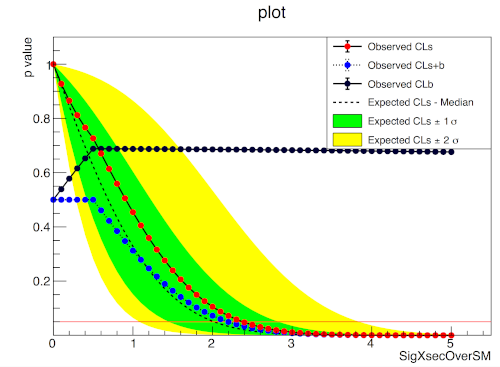Fork me on GitHub# pure-python fitting/limit-setting/interval estimation HistFactory-style¶The HistFactory p.d.f. template [CERN-OPEN-2012-016] is per-se independent of its implementation in ROOT and sometimes, it’s useful to be able to run statistical analysis outside of ROOT, RooFit, RooStats framework.

This repo is a pure-python implementation of that statistical model for multi-bin histogram-based analysis and its interval estimation is based on the asymptotic formulas of “Asymptotic formulae for likelihood-based tests of new physics” [arXiv:1007.1727]. The aim is also to support modern computational graph libraries such as PyTorch and TensorFlow in order to make use of features such as autodifferentiation and GPU acceleration.

## Hello World¶

>>> import pyhf
>>> pdf = pyhf.simplemodels.hepdata_like(signal_data=[12.0, 11.0], bkg_data=[50.0, 52.0], bkg_uncerts=[3.0, 7.0])
>>> CLs_obs, CLs_exp = pyhf.infer.hypotest(1.0, [51, 48] + pdf.config.auxdata, pdf, return_expected=True)
>>> print('Observed: {}, Expected: {}'.format(CLs_obs, CLs_exp))
Observed: [0.05290116], Expected: [0.06445521]


## What does it support¶

Implemented variations:

• [x] HistoSys

• [x] OverallSys

• [x] ShapeSys

• [x] NormFactor

• [x] Multiple Channels

• [x] Import from XML + ROOT via uproot

• [x] ShapeFactor

• [x] StatError

• [x] Lumi Uncertainty

Computational Backends:

• [x] NumPy

• [x] PyTorch

• [x] TensorFlow

• [x] JAX

Available Optimizers

NumPy

Tensorflow

PyTorch

SLSQP (scipy.optimize)

Newton’s Method (autodiff)

Newton’s Method (autodiff)

MINUIT (iminuit)

.

.

## Todo¶

• [ ] StatConfig

• [ ] Non-asymptotic calculators

results obtained from this package are validated against output computed from HistFactory workspaces

## A one bin example¶

nobs = 55, b = 50, db = 7, nom_sig = 10.## A two bin example¶

bin 1: nobs = 100, b = 100, db = 15., nom_sig = 30.
bin 2: nobs = 145, b = 150, db = 20., nom_sig = 45.## Installation¶

To install pyhf from PyPI with the NumPy backend run

pip install pyhf


and to install pyhf with additional backends run

pip install pyhf[tensorflow,torch]


or a subset of the options.

To uninstall run

pip uninstall pyhf


## Authors¶

Please check the contribution statistics for a list of contributors